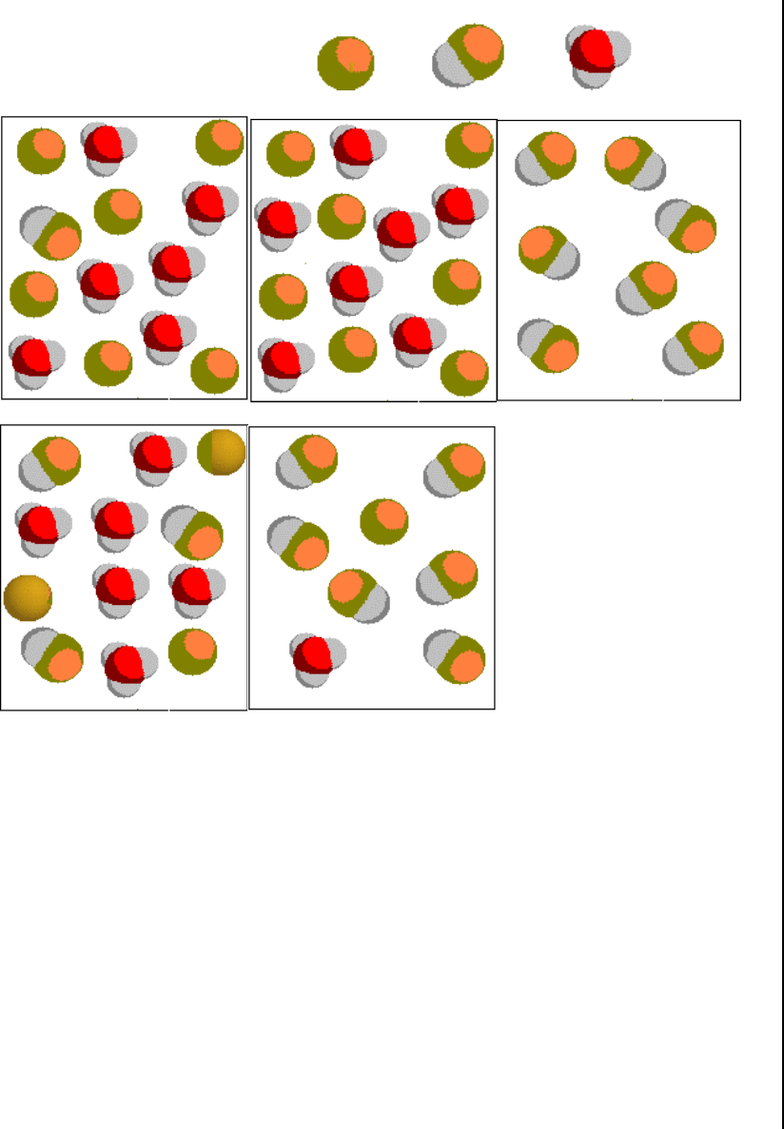# Exam 2

89 views5 pages
School
ASU
Department
Chemistry
Course
CHM 114
ProfessorCHM-115-A Sample Exam 2
Copyright by James P. Birk 1
1. The conjugate acid of hydrogen carbonate ion, HCO3
, in aqueous solution is
a. H2O
b. H3O+
c. H2CO3
d. CO3
2
e. OH
2. The pOH of a 0.150 M HI aqueous solution equals
a. 0.15
b. 0.823
c. 7.00
d. 13.2
e. 13.9
3. What oxide, when dissolved in water, produces a basic solution?
b. oxide of carbon
c. oxide of sulfur
d. oxide of nitrogen
e. oxide of arsenic
4. Which of the following weak acids has the anion that is the strongest base?
a. CH3CO2H, Ka = 1.7 x 10 5
b. HF, Ka = 6.8 x 10 4
c. HCO2H, Ka = 1.8 x 10 4
d. HCN, Ka = 4.8 x 10 10
e. HOCl, Ka = 3.0 x 10 8
5. A polyprotic acid, H5Y, dissociates in five consecutive steps. Which is the strongest
strongest strongest
strongest acid?
a. HY4
b. H2Y3
c. H3Y2
d. H4Y
e. H5Y
6. Which of the following is true
truetrue
true concerning a 0.10 M solution of the weak acid HF?
a. pH < 7.00
b. pH > 7.00
c. [OH ] > [H+]
d. [H+] = [OH]
e. the concentration of F is 0.10 M
7. Calculate the pH of a 0.010 M HOCN solution. Ka = 2.19 x 10 4.
a. 2.48
b. 2.82
c. 3.19
d. 4.66
e. 7.00
1. c
2. d
3. a
4. d
5. e
6. a
7. b
Unlock document

This preview shows pages 1-2 of the document.
Unlock all 5 pages and 3 million more documents.CHM-115-A Sample Exam 2
Copyright by James P. Birk 2
8. Which of the following pictures best represents a weak acid (with Ka << 1) in aqueous solution?
(H2O molecules are not represented.) = A ; = HA; = H3O+
a. b. c.
d. e.
9. Which of the following aqueous solutions could form a buffer when mixed in appropriate
amounts?
a. HNO3 and HCl
b. HCN and KCl
c. KCN and NaCl
d. NaCN and HCN
e. HCl and NaCl
10. A 0.10 M KF solution is basic. Suppose that 0.1 mole of KCN is added to this solution, with no
volume change. What change in pH is to be expected? Ka = 4.0 x 104 for HF and Ka = 4.9 x 1010
for HCN.
a. the pH will rise because KCN is a strong base
b. the pH will rise because KCN is a weak base
c. no change in pH
d. the pH will drop drastically because both KF and KCN are bases
e. the pH will drop because both HF and HCN are acids
8. e
9. d
10. a
Unlock document

This preview shows pages 1-2 of the document.
Unlock all 5 pages and 3 million more documents.

## Document Summary

Chm-115-a: the conjugate acid of hydrogen carbonate ion, hco3. , in aqueous solution is: h2o, h3o, h2co3. Ka = 2. 19 x 10 4: hy. Answers: c, d, a, d, e, a, b. Sample exam 2: which of the following pictures best represents a weak acid (with ka << 1) in aqueous solution? (h2o molecules are not represented. ) Suppose that 0. 1 mole of kcn is added to this solution, with no volume change. 9. 40: calculate the molar solubility of baco3 in water. Ksp = 8. 1 x 10 9: you have a saturated solution of pbi2 in water. Sample exam 2: the drawing shows the distribution of various species in a mixture of hg2+ and cl . (aq) + bi3+(aq: 1, 2, 3, 4, 5, some metallic elements are arranged at the right in an activity series. Select a substance that will reduce ag+ to ag, but will not reduce.